Visualizing af::array with Forge

Arrayfire as a library aims to provide a robust and easy to use platform for high-performance, parallel and GPU computing.

The goal of Forge, an OpenGL visualization library, is to provide equally robust visualizations that are interoperable between Arrayfire data-structures and an OpenGL context.

Arrayfire provides wrapper functions that are designed to be a simple interface to visualize af::arrays. These functions perform various interop tasks. One in particular is that instead of wasting time copying and reformatting data from the GPU to the host and back to the GPU, we can draw directly from GPU-data to GPU-framebuffers! This saves 2 memory copies.

Visualizations can be manipulated with a mouse. The following actions are available:

• zoom (Alt + Mouse Left Click, move up & down)
• pan (Just left click and drag)
• rotation (Mouse right click - track ball rotation).

Let's see exactly what visuals we can illuminate with forge and how Arrayfire anneals the data between the two libraries.

# Setup

Before we can call Forge functions, we need to set up the related "canvas" classes. Forge functions are tied to the af::Window class. First let's create a window:

const static int width = 512, height = 512;
af::Window window(width, height, "2D plot example title");
do{
//drawing functions here
} while( !window.close() );
Window object to render af::arrays.
Definition: graphics.h:37

We also added a drawing loop, so now we can use Forge's drawing functions to draw to the window. The drawing functions present in Forge are listed below.

# Rendering Functions

Documentation for rendering functions can be found here.

## Image

The af::Window::image() function can be used to plot grayscale or color images. To plot a grayscale image a 2d array should be passed into the function. Let's see this on a static noise example:

array img = constant(0, width, height); //make a black image
array random = randu(width, height); //make random [0,1] distribution
img(random > 0.5) = 1; //set all pixels where distribution > 0.5 to white
window.image(img);
array constant(T val, const dim4 &dims, const dtype ty=(af_dtype) dtype_traits< T >::ctype)
static af::array array(af::dim4 idims, cl_mem buf, af::dtype type, bool retain=false)
Create an af::array object from an OpenCL cl_mem buffer.
Definition: opencl.h:327
AFAPI array randu(const dim4 &dims, const dtype ty, randomEngine &r)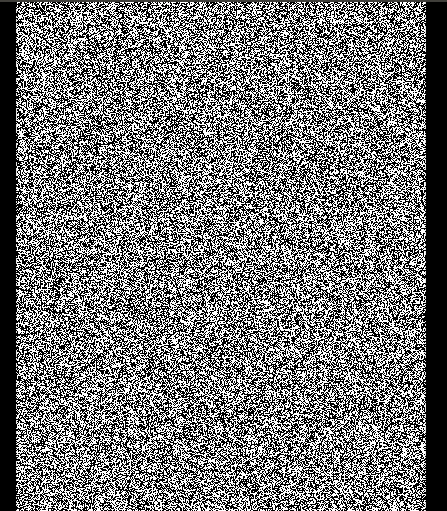Tweaking the previous example by giving our image a depth of 3 for the RGB values allows us to generate colorful noise:

array img = 255 * randu(width, height, 3); //make random [0, 255] distribution
window.image( img.as(u8) );
@ u8
8-bit unsigned integral values
Definition: defines.h:218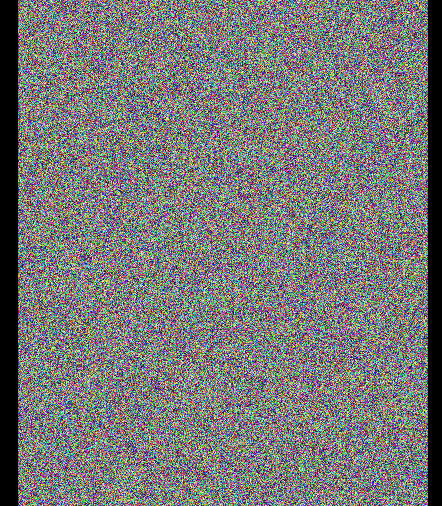Note that Forge automatically handles any af::array type passed from Arrayfire. In the first example we passed in an image of floats in the range [0, 1]. In the last example we cast our array to an unsigned byte array with the range [0, 255]. The type-handling properties are consistent for all Forge drawing functions.

## Plot

The af::Window::plot() function visualizes an array as a 2d-line plot. Let's see a simple example:

array X = seq(-af::Pi, af::Pi, 0.01);
array Y = sin(X);
window.plot(X, Y);
AFAPI array sin(const array &in)
C++ Interface for sin.
AFAPI const double Pi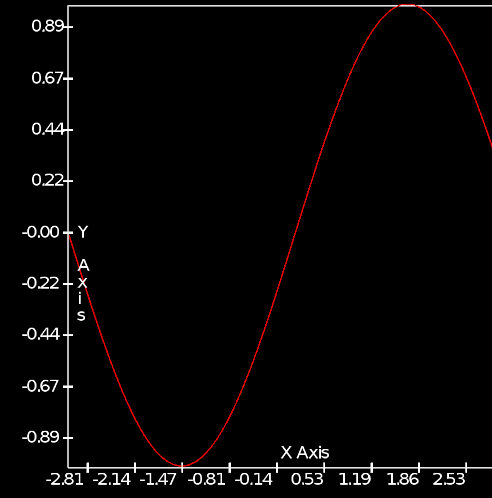The plot function has the signature:

void plot( const array &X, const array &Y, const char * const title = NULL );

Both the x and y coordinates of the points are required to plot. This allows for non-uniform, or parametric plots:

array t = seq(0, 100, 0.01);
array X = sin(t) * (exp(cos(t)) - 2 * cos(4 * t) - pow(sin(t / 12), 5));
array Y = cos(t) * (exp(cos(t)) - 2 * cos(4 * t) - pow(sin(t / 12), 5));
window.plot(X, Y);
AFAPI array cos(const array &in)
C++ Interface for cos.
AFAPI array exp(const array &in)
C++ Interface for exponential of an array.
AFAPI array pow(const array &lhs, const array &rhs)
C++ Interface for power.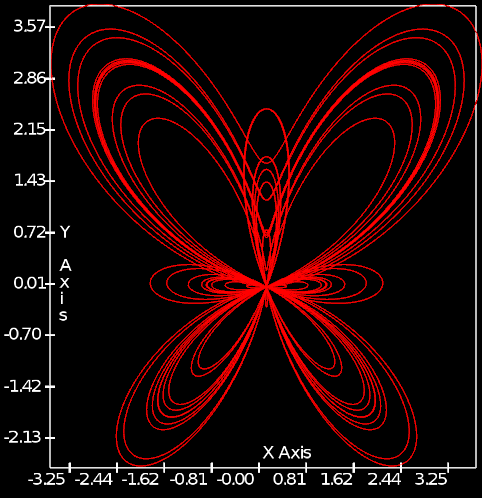## Plot3

The af::Window::plot3() function will plot a curve in 3d-space. Its signature is:

void plot3 (const array &in, const char * title = NULL);

The input array expects xyz-triplets in sequential order. The points can be in a flattened one dimensional (3n x 1) array, or in one of the (3 x n), (n x 3) matrix forms.

array Z = seq(0.1f, 10.f, 0.01);
array Y = sin(10 * Z) / Z;
array X = cos(10 * Z) / Z;
array Pts = join(1, X, Y, Z);
//Pts can be passed in as a matrix in the from n x 3, 3 x n
//or in the flattened xyz-triplet array with size 3n x 1
window.plot3(Pts);
//both of the following are equally valid
//window.plot3(transpose(Pts));
//window.plot3(flat(Pts));
AFAPI array join(const int dim, const array &first, const array &second)
Join 2 arrays along dim.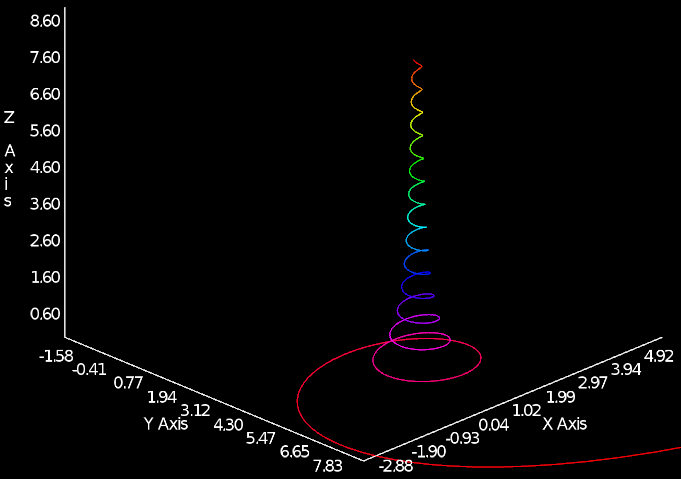## Histogram

The af::Window::hist() function renders an input array as a histogram. In our example, the input array will be created with Arrayfire's histogram() function, which actually counts and bins each sample. The output from histogram() can directly be fed into the af::Window::hist() rendering function.

const int BINS = 128; SAMPLES = 9162;
array norm = randn(SAMPLES);
array hist_arr = histogram(norm, BINS);
win.hist(hist_arr, 0, BINS);
AFAPI array histogram(const array &in, const unsigned nbins, const double minval, const double maxval)
C++ Interface for histogram.
AFAPI double norm(const array &in, const normType type=AF_NORM_EUCLID, const double p=1, const double q=1)
C++ Interface for norm of a matrix.
AFAPI array randn(const dim4 &dims, const dtype ty, randomEngine &r)

In addition to the histogram array with the number of samples in each bin, the af::Window::hist() function takes two additional parameters – the minimum and maximum values of all datapoints in the histogram array. This effectively sets the range of the binned data. The full signature of af::Window::hist() is:

void hist(const array & X, const double minval, const double maxval, const char * const title = NULL);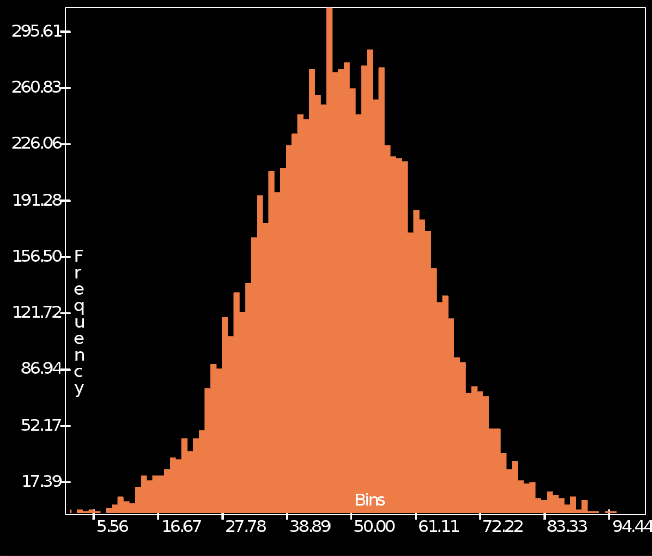## Surface

The af::Window::surface() function will plot af::arrays as a 3d surface.

array Z = randu(21, 21);
window.surface(Z, "Random Surface"); //equal to next function call
//window.surface( seq(-1, 1, 0.1), seq(-1, 1, 0.1), Z, "Random Surface");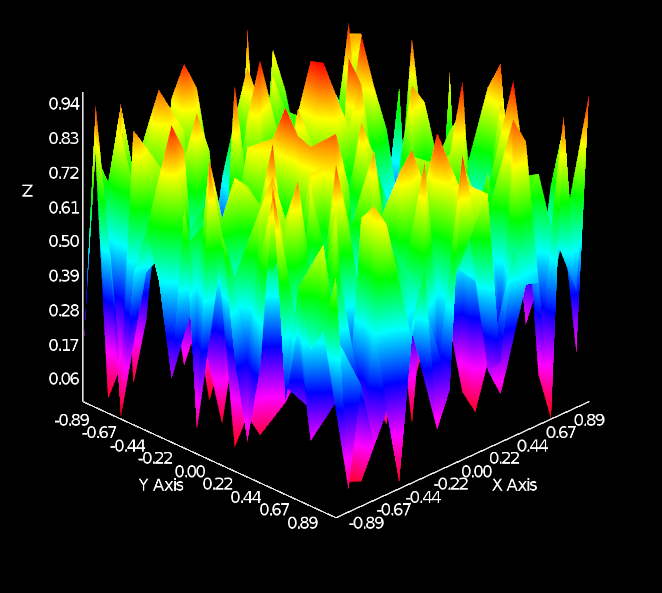There are two overloads for the af::Window::surface() function:

void surface (const array & S, const char * const title ) // Accepts a 2d matrix with the z values of the surface

void surface (const array &xVals, const array &yVals, const array &S, const char * const title) // accepts additional vectors that define the x,y coordinates for the surface points.

The second overload has two options for the x, y coordinate vectors. Assuming a surface grid of size m x n:

1. Short vectors defining the spacing along each axis. Vectors will have sizes m x 1 and n x 1.
2. Vectors containing the coordinates of each and every point. Each of the vectors will have length mn x 1. This can be used for completely non-uniform or parametric surfaces.

# Conclusion

There is a fairly comprehensive collection of methods to visualize data in Arrayfire. Thanks to the high-performance gpu plotting library Forge, the provided Arrayfire functions not only make visualizations as simple as possible, but keep them as robust as the rest of the Arrayfire library.